﻿ houghton biology site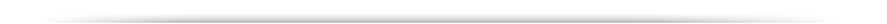To Recap Aspects of Mendelian Genetics that we went over yesterday....test cross?

Ratios: 3:1 and 1:1 (test cross) for monihybrid crosses and 9:3:3:1 for dihybrid crosses.

Extension of Mendelian Genetics:-

 Incomplete dominance: eg. Four o' clocks, carnations. BLENDING 1:2:1 Codominance: eg. M and N blood groups on chromosome 4 having specific antigens M and N 1:2:1 but where the heterozygotes (MN) gives rise to a distinct phenotype Multiple alleles ABO blood types, A and B are dominant to O , but A and B codominant to each other Epistasis essentially "eliminates" or masks phenotypic expression of other genes, eg. Labrador dogs fur colour, albinoism in mice Lethal alleles eg. Yellow colouration in mice fur. 2 : 1 . Pleiotropy(?) Several genes / same character Coat colour in mammals, eg. mice A (agouti), B (black/brown), C, (colour) D (intensity), S. (distribution) genes Complementary gene activity eg. Pea plants, purple colouration. 9 : 7 Duplicate gene activity eg. Shepherd's purse, Round over narrow fruits, where both A 1 - and A 2 - can cause heart shape 15 : 1 Recessive suppression eg. Case of purple eye colour in the fruit fly. 13 : 3

Complementary gene activity, occurs when two distinct genes affect the same phenotype in a complementary way, whereby the presence of BOTH dominant alleles are required to give a particular phenotype that is distinct from either being present by itself.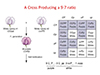Recessive Suppression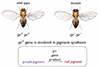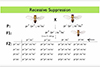Discovery of Linkage: T. S. Morgan (1909) working on non-autosomal and sex chromosome -linked genes of Drosophila melanogaster -the commmon fruit fly.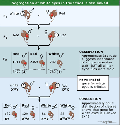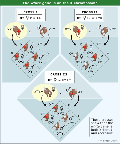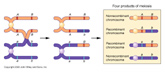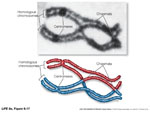While the X and Y  chromosomes normally behave according to the laws of meiotic cell division (and thus according to Mendelian genetics) the ability to "visualize" through genetic crosses the presence of each of the alleles that is located on either of the X-chromosomes of a mother directly challenges the ubiquity of Mendel's 2nd law).

Remember, that ... to equate Mendel with meiosis we had to invoke the role of chiasmata occuring between two gene loci on adjacent chromatids in paired chromosomes during prophase I of meiosis.   This CANNOT occur for X and Y chromosomes,

But what about ALL the genes on the remainder of the non sex chromosomes or "autosomal" chromosomes.

Again, I glibbly stated yesterday that, if the probability of a chiasma forming between two genes is "one" (i.e. a certainty), then the assortment of alleles would, in essence, be the same as if they were on separate chromosomes (i.e. random).

But Morgan went further. He analyzed the likelihood of the hereditary outcomes of TWO genes "white eye" and "miniature wing". which he knew (from previous studies) were on the same X chromosome,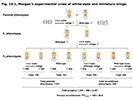Morgan then went on to analyze other genes and demonstrated that some genes -other than the ones that he had tracked to the X chromosome- also failed to behave according to Mendelian expectations, and appeared to retain some of the gene association traits that were present to one or the other of the parents.

Two such traits (not on the X chromosome): purple eye colour (pr, purple and pr+, red) and vestigial wing (vg, vestigial and vg+ normal).

cross       pr+ pr+ and vg+ vg+  x   pr pr and vg vg

giving an -> F1 gen. of      pr+ pr / vg+ vg.

He then test crossed the females of these flies with male flies that were double recessive (i.e. recessive for both traits)

giving an -> F2 gen. of.......

 pr+ vg+ 1339 pr vg 1195 pr+ vg 151 pr vg+ 154

.......demonstrating an apparent association of Wild-Type and mutant phenotypes, or "gene coupling"

Morgan then undertook a different cross using a

pr+ pr+and vg vg with a pr pr and vg+ vg+male

giving a similar -> F1   gen. of pr+ pr / vg+ vg .

Again he then took the females of these flies with male flies that were double recessive

giving an -> F2 gen. of.......

 pr+ vg+ 157 pr vg 146 pr+ vg 965 pr vg+ 1067

..........seemingly demonstrating an abnormally overt lack of association or "gene repulsion", but having the same gene associations as the original parents.

Morgan explained the anomalies by proposing that the two loci were present and "linked" on the same chromosome.

He went further to suggest that such linkage was apparent because of the "proximity" of genes to each other on the chromosome, which modifed the possibility of chiasmata being able to completely randomize their assortment in to the gametes.......remember those "mobile nodes" or chiasmata???  When do they occur?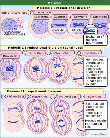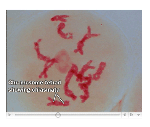Meiosis.

Additional, physical evidence for these crossover events came much later, and were defined by Creighton and McClintock (1931) while trying to understand the gentic assortment of two genes in corn: Waxy [Wx] (starchy ) and Colour [C] (colourless). These two genes were found to be linked on chromosome #9, and their crossover phenotypes were always reflected in the physical aberrations at the extremities on each of the chromosomes.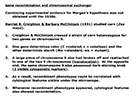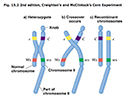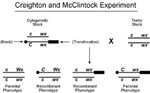Morgan went further.  He proposed that the frequency of such cross-overevents (occurring between two gene pairs) was a function of the genetic distance between the two loci..., BUT ONLY as the gene pairs were relatively quite close to each other on the chromosome.

He thus defined the unit of genetic distance as being:

one crossover event/100 products of meiosis = one map unit or 1 centiMorgan (cM)

If this were to be the case, then perhaps the apparent association or linkage could be used to define the order of the location of genes that were relatively close together onthe chromosome

The answer to this idea was Yes, because the probability of crossing over occuring between two loci that were relatively close to each other on the same chromosome appeared to be additive.

Thus, in the following linkage map the probability of a crossing over event pccuring between Y and M would be expected to be 35%.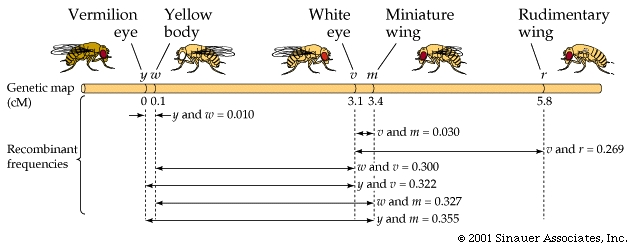Y-----W- -------------------------------------------M 0.5 34.5

One of Morgan's students, Sturtevant, quickly realized that such information could be used to map genes.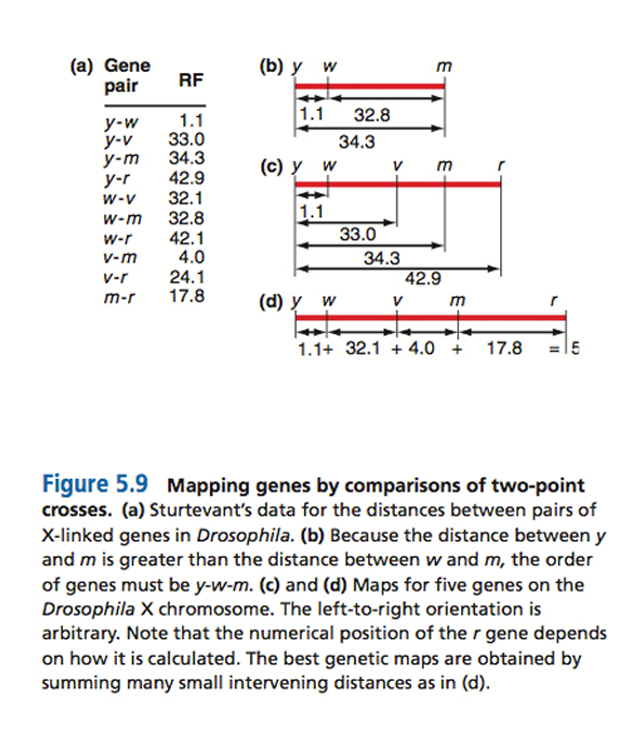Three point test crosses. Mathematically, two independent events happening at the same time-

However, to observe these traits.....

1. Organism producing the crossover gametes must be heterozygous for all three traits.

2. The cross must be constructed so that each of the phenotypic traits must reflect a change in the genotype at each locus.

3. A sufficient number of offspring must be obtained to get statistically viable results.

In determining the gene order among three genes. You will need to know how this can be achieved!!

eg.

F1:

 a b c A B C

This is equivalent to the required heterozygotic parental generation for all three traits.

Remember that this is the simplest case where all the wild-type alleles are dominant and, at least in this case, on the same chromosome.  Correspondingly, ALL the mutant alleles are iniitially on the other.

In the next generation these triple heterozygotes can be test- crossed with triply recessive testers (???)............

In so doing, any "crossing-over" events that have occurred in the formation of the gametes will show through against the triply recessive pairing.

F2: listed as gametic genotypes...Crossover Types

 a b c = 230 = 467 = 42.1% = NCO A B C = 237 a B C = 82 = 161 = 14.5% = SCO A b c = 79 a b C = 200 = 395 = 35.6% = SCO A B c = 195 a B c = 44 = 86 = 7.8% = DCO A b C = 42

Thus, some vindication of the underlying linkage principle... but this all assumes the premise that the gene order is a b c

Another example: Cross of X/X y/y z/z with x/x Y/Y Z/Z is, however, not appear to behave is such an overtly simple manner, = -as there is now what is termed a mixed "association" of =Wild-Type and mutant alleles, giving:

F1

 X y z x Y Z

Now if this triply heterozygotic strain were to be test-crossed with a triple recessive...! yielding 1448 total offspring

 X y z = 580 = 1172 = 80.9% = NCO x Y Z = 592 X y Z = 45 = 85 = 5.9% = SCO x Y z = 40 X Y z = 89 = 183 = 12.6% = SCO x y Z = 94 X Y Z = 3 = 8 = 0.5% = DCO x y z = 5

But these problem only arise, however, if we assume the genes are in alphabetical order; that, with respect to x and y, the parent types are "X y z " and "x Y Z"

If they are not, then:

The first question would be....... What is the gene order?  We find this out by looking at theNCO's and the DCO's.

 X y z x Y Z

What would be the gene order that gives "X Y Z" as a DOUBLE cross over

The answer is ...."y     x      z".

Now both the single cross overs (SCO) and double crossovers DCO make sense.

It also allows us toe guage the recombinant distance between the outside genes pairs of y and zby adding up the SCO values between y --- x and x --- z = 268 / 1448 =18.5% to be the recombination frequency value (RF) between the outside genes pairs of y and z

If one does the same thing for the other two sets of gene pairs then one can obtain recombination frequency values (RF values) of 13.1%  for y and x, and 6.4% for x and z, respectively

But 13.1 + 6.4 = 19.5%, which is a little higher than the previously calculated 18.5%

How can that be??

-because the DCO's are, as the name suggest, double cross overs -BOTH of which need to be considered when evaluating crossover frequency.

The Second question: Are the relative rates of SCO's and DCO's consistent? If not..... ?

Morgan's student, Sturtevant, observed this phenomenon in a number of his crosses, and rationally understood that frequency of recombinants increased with increasing distance, and that it was more accurate to add the intervening distances from multiple small intervals, rather than rely on a single cross between two fairly distant markers to determine the map distance.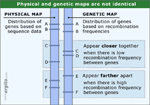This is not always the case, however.  The basic assumption being that the formation of a chiasma is a physical event and therefore as you analyze genes which are closer and closer together, there has to be a physical limit where stearic hindrance in the formation of one synapse (at the synaptonemal complex) begins to interfere with the formation of the next.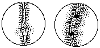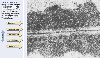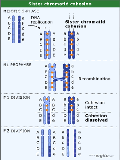To measure interference, we first calculate what is termed the coefficient of coincidence (c.o.c.), which essentially defines the ratio of observed to expected double recombinants.

Interference is then calculated as 1 (unity) - c.o.c.

Along these same lines, assuming that there is enough room between the position of different genes residing on the same chromosome, if you can have one crossover between two loci residing on two non-sister chromatids then what's to stop there being more than one?

Again, as we have just discussed, if you have three loci that are close together, then you can perform the proverbial three point test cross, which ought to enhance your accuracy somewhat (and at the very least establish a gene order).

But what do you do if you don't have an appropriately defined third locus lying in between your two loci of choice? Moreover, what happens if you haven't got all the appropriate mutant strains... ?? Accurate determination of the true recombination distance would be compromised

Given that it's all a matter of probabilities.... refer to statistics.

Use of mathematical equation that was developed to predict the probability of random events....Poisson distribution.

 ƒ(a) = e-m [ ma] [  a! ]

where

a = the class of variables that you want to observe (the number of crossovers),

m = the mean number of items that you expect to get in your variations,

e = the base of the natural log

and ! = factorial function

Recombination frequency (RF) and the "ultimate mapping unit"....(m) can now be squeezed into this equation

In an assortment of genes where there is at least one crossover, we can contrast among all the different events.

The RF (frequency of recombinants) can be deduced empirically.

RF (if there are no crossovers) = 0

RF (if one crossover) recombinants ought to make up 50% of the possible products.

RF (if 2 crossovers) recombinants also appear to be pretty much the same....~ 50%.

Indeed, it turns out that it really doesn't matter how many recombinants you look for, the number of recombinants make up approximately one half of all the potential products of meiosis.

Therefore, the proportion of meioses with at least one crossover is

Unity (1) minus the fraction of those outcomes that have zero crossovers.

This "fraction" can be deduced through Poissonic logic by taking "a" = 0,

giving the fraction = e-m [m0/0!] = e-m

Thus, RF( recombination frequency) = 0.5 x (1 - e -m ).

which relates mapping units "m" (or the mean number of exchanges) directly to RF.

Now, if we express m as a "percentage fraction of crossovers" in order that we can equate it to centiMorgans (or the official map units) we must convert the value.....

Example: An empirically defined RF between two gene pair locations = 0.275 (27.5%).

plugging this value into the equation

=> 0.275 = 0.5 x (1 - e -m )

=> e-m =   1 - 0.55 = 0.45,

then to get a value for "m" go to a log table (which will always be provided in any test if necessary),

or.....

 Solve for "m" e-m = m = 0.45 0.8

But we are not quite finished yet.!!!!

We still need to convert this value to cM or real map units......

We have previously found that 100% (non-linkage) = 50 cM.

Thus, 50 cM is the highest number of centiMorgans you can get....effectively, therefore, all linked gene pairs at 50cM would behave according to Mendelian Principles, and not be subject to Poisson distributional analysis

Therefore, the true distance between two loci that DO experience linkage (in cM or map units)

=  50 x m cM.

.......50 * 0.8 = 40cM, which (as it turns out) is considerably greater than the original value of 27.5 cM, that was empirically deduced

Having done this a number of times, Geneticists were able to plot this mapping function on to a curve.....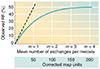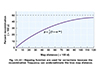OK, so we now have some mathematical appreciation for linkage, and understand how Mendelian principles may and/or may not apply to the genes that are present on the same chromosome, what now?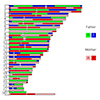We can begin to appreciate how genes can be mapped on a chromosome, simply by applying the rules of linkage that Morgan and his students have presented, and asign positions or genetic loci relative to other genes and physical/genetic features on different chromosomes as a function of their recombination frequencies.  You must not forget, however, that genetic maps do not always equate to physical maps.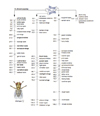It has always been curious to me that the discovery of linkage actually occurred using the sex chromomomes in Drosophila, which are the most dissimilar of all chromosomes and which highlight major unique features of sex-linkage...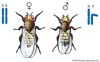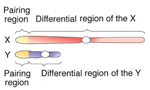The inbility to form chiasmata between homologous chromosomes that occurs in meiosis prophase I can also occur ine what are called Balancer chromosomes, or rearranged chromosomes that can no longer line up appropriately in prophase 1 to allow for chiasmata to form. These balancer chromosomes can be constructed synthetically and/or can occur naturally. Either way they can be used extensively to analyze the inheritance of genetic traits across generations without the interference of chiasmatic crossing over events exchanging information between similar reions of chromosomes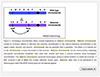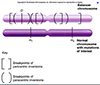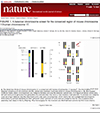There are some variables of gene "associations" that result from gene linkage and chromosomal misbehaviour.

Meiotic Nondysjunction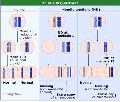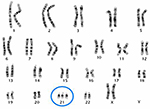Mitotic Nondysjunction.

(a) Calvin Bridges noticed that a strain of flies M M+ (heterozygous for "bristle" configuration), sometimes expressed itself as a "local variant" of the dominant M allele (slender bristle) in which a region of the fly’s body exhibited wild-type (M+) bristle characteristics.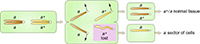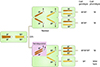He attributed this phenomenon to a genetic event termed mitotic nondysjunction, where one half of the resulting progeny have only three copies of the chromosome (resulting from an aberrant mitotic division) and the other cell has only one.

Sometimes such a localized phenotypic variation can occur as a result of chromosome loss during mitosis of a somatic or non-germ-line cell.

Either way, the local variation was, and is, thought to result from a catastrophic loss or abnormal migration of whole chromosomes.

Segregation at the phenotypic level, i.e. heterozygotes exhibiting localized "patches" of variant phenotypes.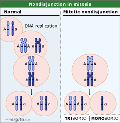(b) In 1936 Curt Sterns (another student of Morgan's working on D. melanogaster) undertook a cross of two other sex-linked traits y (yellow hair) and sg (singed hair).
y+ sn / y+ sn (singe-haired female) with y sn+ / Y (yellow singe-haired male)

Not too surprisingly the female progeny were mostly Wild-Type with grey bodies and normal bristles y+ sn / y sn+

Occasionally, however, Stern noticed that there were some localized twin spots of apparently "coupled" yellow and singed hair (y sn) phenotypes, which occurred as twin spots too often as to be mere coincidental juxtapositioning. How might these be explained?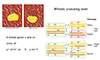He accounted for this by asserting the phenomenon of mitotic recombination, i.e. the chance occurrence of a crossing over event occurring between two homologous chromosomes during MITOSIS, whereby the event occurs between the two gene loci and the centromere.

N.B. Don't forget that diploid cells give rise to diploid progeny in mitotic divisions!!

Sterns' work was the first example of "mosaicism", which is the presene of two or more populations of cells with different genotypes. It was the birth of what is now a significnt branch of molecular genetics called "mosaic analysis", which is one of the major tools used today to analyze the effects of lethal or "specifying" genes in a number of organisms, by being able to compare the genetic heritage of two cell lines -with the only difference between the two being a somatic aberration, that can often be induced.

Additional concerns with genetic exchange through crossing over.Consider a pair of homologous chromosomes prior to the MI division which contain a single crossover in the interval between markers B and D on chromatids 2 and 3, as shown in the Figure below. The outcome of the single crossover is two chromatids carrying a recombination of the phenotypic markers associated with the B and D genes. Now, consider that a second crossover occurs in this same interval. There are four ways this could occur, and each of these is equally probable.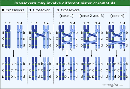• (Case 1):   As we have alrady dealt with.  The second crossover could occur again between chromatids 2 and 3, restoring the original configuration of markers B and D. If the investigator were only analyzing recombination of B and D, then the double crossover involving two strands would appear as though there had been no crossover at all: it would be indistinguishable from the 0 crossover class. Only if the investigator follows marker C in this example would the double crossover be apparent, providing a reciprocal recombination event of B-c-D and b-C-d.

• (Cases 2 and 3): The second crossover could involve only one of the original chromatids. The first crossover involved chromatids 2 and 3, so the second crossover could involve 1 and 3, or 2 and 4. (Recall that the meiotic crossover predominantly occurs between homologous chromosomes, and not between sister chromatids. Therefore, it is unlikely to occur between 1 and 2, or between 3 and 4.) This double crossover, involving three strands, gives the same recombination of the phenotypic markers as in the single crossover (only two chromatids show a recombination between B and D) and can only be distinguished from the single crossover class by analysis of the C marker.

• (Case 4): Finally, the second crossover could occur between chromatids 1 and 4, neither of which were involved in the first crossover. This case is unique, since it results in four recombinant chromosomes and no wild type associations. This feature is particularly powerful in identifying the frequency of double crossovers: this class can often be identified unambiguously, and represents 1/4 * the total number of double crossovers. Using tetrad analysis to determine gene-gene linkage for an example).

How do we analyze for this and asure ourselves that each non-sister chrmatid has an equal chance of crossing over with each of the other paired non-sister chromatids.

Yeast and fungi.

S. cerevisiae: and the potential POWER of yeast genetics -provides relatively immediate proof of all types of chiasmata and their consequences. Use of yeast and other similar fungi have many distinct advantages ...S. cerevisiae can grow mitotically in a stable fashion as either a haploid (with one copy of each chromosome) or a diploid (with two copies of each chromosome).  In essence, therefore, the consequences of meiosis can be "harvested" through analysis of both states, and the direct products of meoiotic events can be analyzed.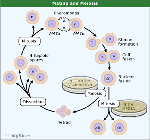Genetic Orderliness in Ordered and Unordered Tetrad analyses

Indeed, all fungi and algae form "haploid" spores, that contain 4 or 8 spores, resulting from a meiotic division.

These can be "ordered" or "unordered" within their respective asci (spore bags).

Linear tetrad analyses allows for "centromere mapping" or the distance from any given locus to the centromere (again using recombination as a tool to determine distance).

In choosing two linked genotypes that are easily discernible (phenotypes) this can give rise to a simple analysis of meiotic function.

e.g.                   a       b          x          a+        b+

Three different assortment options no matter whether the two genes are linked or not

 1 2 3 a b a b+ a b a b a b+ a b+ a+ b+ a+ b a+ b a+ b+ a+ b a+ b+
Parental Ditype (PD).......Non-Parental Ditype(NPD) ..........Tetra-Type (TT)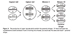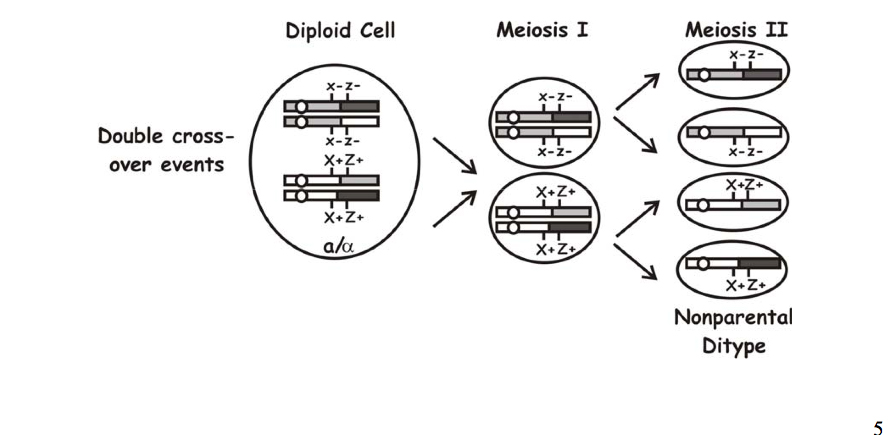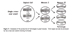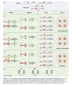The power of tetrad analysis comes from the fact that all four meiotic products are contained within a single ascus.

The most fundamental test by tetrad analysis is the one for single-gene segregation. This can determine whether a mutant phenotype is caused by a mutation in a single gene or not. If a single gene is the cause, the mutation should segregate within the tetrads in a predictable, Mendelian fashion for a single gene.

The ability to use tetrad analysis to map genes derives directly from the special information that can be obtained from having all four meiotic products in an ascus. As described below, this allows us to make and test specific predictions regarding the segregation patterns that will occur depending on whether two genes are linked or unlinked.

• A parental ditype (PD) is a tetrad in which two genetic markers are segregating, and two spores have one parental genotype and two spores have the other parental genotype.

• A nonparental ditype (NPD) is a tetrad in which two genetic markers are segregating, and two spores have one nonparental genotype and two spores have the other nonparental genotype.

The first class of tetrad is that in which the two parental sets of markers segregate away from each other at meiosis I.Meiosis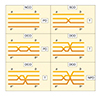The resulting tetrads have two spores bearing the genotype of one parent (HIS4 - trp1) and the other two spores bearing the genotype of the other parent (his4 - TRP1).

This class of tetrad, having the two classes of parental spores, is called a parental ditype (PD),

and this arrangement of spores in the ascus can also be acheived if genes are on different chromosomes or on the same chromosome

The second class of tetrad is that in which segregation at meiosis I occurred in the opposite fashion. The result is a tetrad with two different classes of spores. This class of tetrad, having two classes of nonparental spores, is called a nonparental ditype (NPD)

Similarly, this arrangement of spores in the ascus can be acheived if genes are on different chromosomes or on the same chromosome.

Because segregation at meiosis I is "random" and occurs at equal frequency (in accordance with Mendel's First Law), for two unlinked genes, the frequency of PD tetrads should equal the frequency of NPD tetrads.

This prediction constitutes one of the fundamental tests of genetic linkage in yeast.

Therefore, when testing the possible linkage of two mutations with unknown map positions, the ffundamental question to answer is,

"Does the number of PD's equal the number of NPD's?"

If the answer is YES, the association of mutations (and hence the gene loci) are unlinked.

The third type of tetrad arises as the result of a crossing over, or recombination, between one of the genes and its centromere. Crossing over during yeast meiosis occurs after replication, when there are four chromatids.

For two unlinked genes on different chromosomes the frequency of TT tetrads will depend on the linkage of each gene to its centromere, as it can result from a crossing over of either gene with respect to its centromere. This property can ultimately be used to determine whether a gene is linked to its centromere or not.

For two unlinked genes on the same chromosome (where PD = NPD), a TT tetrad can arise as the result of a crossover between one of the markers and its centromere. (We will see later that TT tetrads can also arise in another way).

---

Now let us consider a different type of linkage, in which two genes are now "LINKED" to each other.

By tetrad analysis we can determine the degree of genetic linkage between such markers.

In the extreme cases of two genes that are completely linked, with no detectable recombination between them, we expect that tetrad analysis will reveal that ALL the tetrads are PD tetrads.

What about cases where the two genes are linked but there is some recombination between them?

In those cases, the number of PD tetrads will be greater than the number of NPD tetrads (PD > NPD). This is because PD tetrads ONLY arise from no recombination.In contrast, for two linked genes, NPD tetrads can also arise by a double crossover between the two linked markers in a three stranded exchange). As for centromere linkage, in the case of closely linked genes, we can calculate the degree of linkage based on the frequencies of the different types of tetrads produced.
..

Recombination (SCO's and DCO's) between linked genes can also produce PD, NPD, and TT tetrads.

-Recall that for two unlinked genes, when PD = NPD, TT tetrads can also arise by a crossover between a gene and its centromere.

However, in the case of linked genes, when PD > NPD, TT tetrads can arise by a single crossover between the two genes and also by two of the four types of double crossovers, as shown in the multiple crossover figure.It is, therefore, possible to relate T(etraTypes) and NPD' s (non-parental ditypes) to the potential single crossover events (SCO's) and double crossover events (DCO's).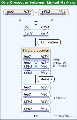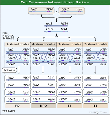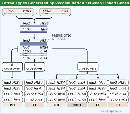With "linked" genes there is only ONE way to obtain an NPD -through a double cross over.

Thus, with respect to the NPD’s .......... the number of DCO's obtained = 4NPD

In a similar way, SCO can be written as being = T - 2NPD, as there are three ways to get a Tetratype (2 of which are DCO's).

Now, from a previous understanding of crossover frequencies

NCO = 1(or unity) - (SCO + DCO)

(assume that triple, quadruple crosses etc can generally be ignored as having less than a significant affect upon the end results)

let m = the mean no. of crossovers, thus m = SCO + 2 x DCO (as the actual number of crossovers in a double cross is 2).

substitute appropriate values:

=> m = (T-2NPD) + 2(4NPD) = T + 6NPD

then (as always) one needs to convert to cM by multiplying by 50 =>

now RF (in cM) = 50 (T + 6NPD)

Chromatid interference.

Note: The theoretically maximal value for RF being 50 can only be true if and when there is NO chromatid interference.Consequently, if you use the formula RF = 50 (T+6NPD) (from the above definition) then the occurrence of chromatid interference might conceivably overestimate the RF value, which could therefore (in practice) actually exceed 50%!!!!!

Centromere mapping. While unordered tetrads allow for a rapid and relatively simple evaluation of recombination frequencies (and therefore genetic linkage of proximal genes present on the same chromosome, analysis of ordered tetrads (or octads) allows for an additional evaluation of the proximity of genes to the centromere.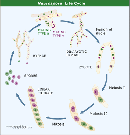Neurospera crassa or Sordaria sp. are examples of fungi that gives rise to an ordered arrangement of its spores within their respective ascospore. Specific variations in the location of given trait within the ascospore leaves a tell-tale assortment of an allele that is due to a crossing over event between the centromere and a given gene locus. In a similar manner to regular crossing over phenomena the distance between the centromere and a gene can thus be estimated by analyzing the frequency of "second division" segregation within the ordered tetrad (octad) and dividing by 2 (as only half of the resulting allele locations are due to this crossing over event).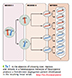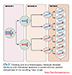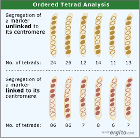e.g. in the case of a crossover between the A locus and the centromere.A a A a A a A a A a A a A a a A a A A a a A a A a A A a a A a A A a a A a A a A A a a A a A A a _____ _____ _____ _____ _____ _____ 126 132 9 11 10 12

Total = 300

The recombinant forms are: 9 + 11 + 10 + 12 = 42  =  (42 / 300) x 100 = 14% are recombinants.

Now divide by two to get cM

=> Locus A therefore lies 7cM from the centromere.

Complications........ it’s always the exceptions that "proves" the rule!

-------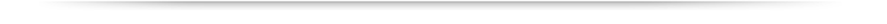Copyright © Department of Biology, Georgia State UniversityView Legal Statement Contact Biology Office: Tel: 404-413-5300
 About Academics Admissions Research Libraries About University Library Campus Life Athletics Alumni